# 2D Shapes

In geometry, 2d shapes and 3d shapes are explained widely to make you understand the different types of objects you come across in real life. These shapes have their own pattern and properties. Depending on many factors, such as angle, sides, length, height, width, area, volume, etc., the shapes can vary. These 2D and 3D shapes have been taught to us since our primary classes. Let us learn various types of two-dimensional shapes here, in this article.

## 2D Shapes Definition

In maths, 2d shapes can be defined as the plane figures that can be drawn on a flat (or plane) surface or a piece of paper. All the 2d shapes have various parameters such as area and perimeter. Some of the 2d shapes contain sides and corners, whereas some have curved boundaries.

## 2D Shapes Names

1. Circle
2. Triangle
3. Square
4. Rectangle
5. Pentagon
6. Octagon

The basic types of 2d shapes are a circle, triangle, square, rectangle, pentagon, quadrilateral, hexagon, octagon, etc. Apart from the circle, all the shapes are considered as polygons, which have sides. A polygon which has all the sides and angles as equal is called a regular polygon. Including the circle, an ellipse is also a non-polygon shape. Both circle and ellipse have a curved shape, whereas the polygons have a closed structure with sides. Now let us discuss some shapes one by one.

### Circle

A circle is a closed 2d figure in which the set of all the points in the plane is equidistant from a given point called “center”. The distance from the center to the outer line of the circle is called a radius. The example of the circle in real life are wheels, pizzas, orbit, etc.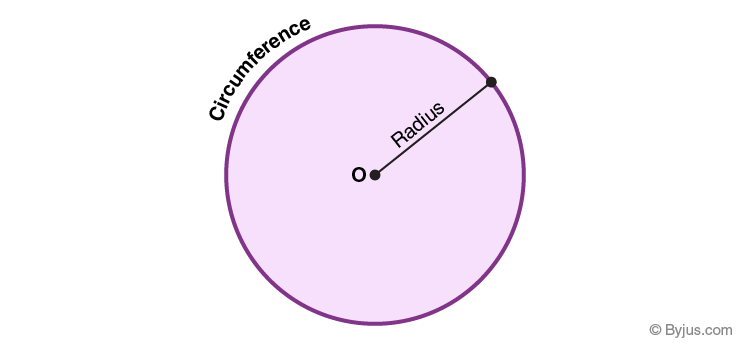### Triangle

A triangle is a three-sided polygon (2d Shape) which has three edges and three vertices. The sum of all the three angles of a triangle is equal to 180°. Pyramids are the best example of a triangle shape. You can also learn the properties of triangle here.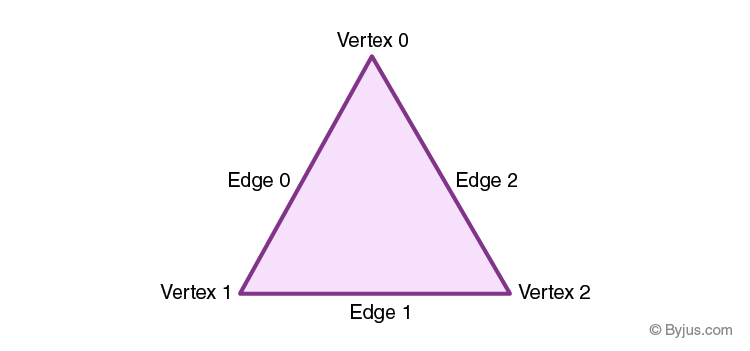### Square

A square is a four-sided polygon (2d Shape), whose four sides are equal in length and all the angles are equal to 90°. It is considered to be a two-dimensional regular quadrilateral. The diagonals of the square also bisect each other at 90°. A wall or a table where all the sides are equal are the examples of square shape.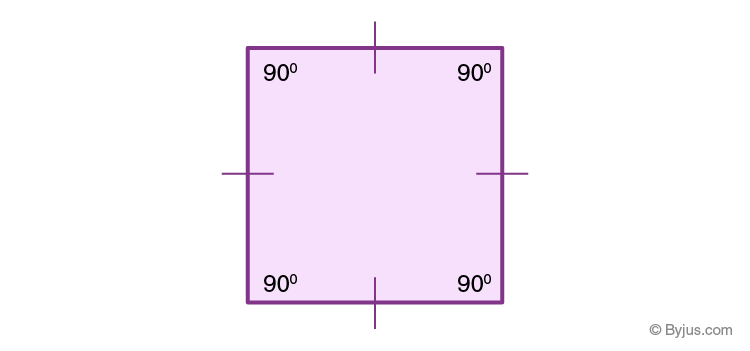### Rectangle

A rectangle is a 2d shape which has four sides, where the opposite sides are equal and parallel to each other. All the angles of a rectangle are equal to 90°. A brick, TV, cardboard, which has length and breadth are examples of the rectangle.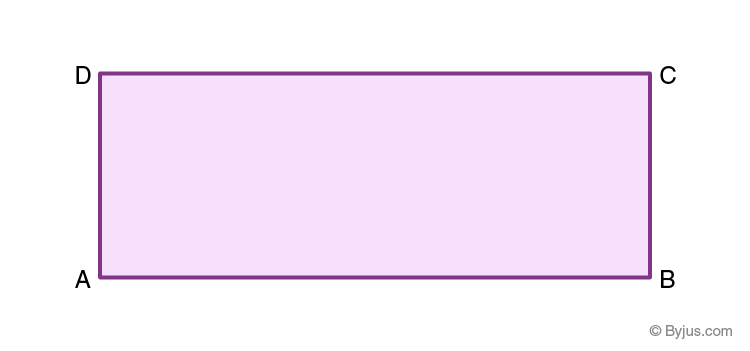### Pentagon

A pentagon is a five-sided polygon (2d Shape), and it can be regular or irregular. In the case of a regular pentagon, each interior angle is equal to 108°, and each exterior angle measures 72°. It has five diagonals. The Pentagon building, which is the headquarters of the US Department of Defense, is a great example of the pentagon shape.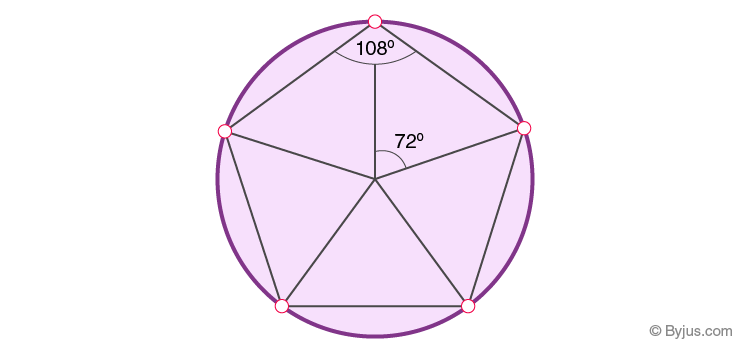### Octagon

An octagon is an eight-sided polygon which can be either regular or irregular. It is a 2d shape which has eight angles. The sum of all the interior angles of an octagon is 1080°. The stop sign board has an octagon shape, which you can see on the roadside.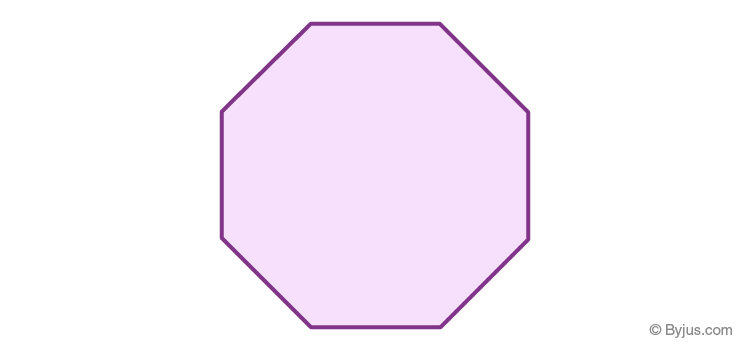### 2D Shapes Properties

Go through the below to learn all the properties of 2D shapes.

 2 D Shapes Properties of 2 D Shapes Square Four equal sides Four equal angles(90°) Four axes of symmetry Rectangle 2 sets of 2 equal sides Four equal angles(90°) Two axes of symmetry Triangle It can have no, 2 or 3 equal sides It can have no, 2 or 3 equal angles It can have up to 2 axes of symmetry Circle Constant diameter and radius The total angle of a circle is equal to 360 degrees Almost infinite axes of symmetry going through the centre pentagon 5 sides (can be equal or unequal) 5 angles (can be equal or unequal) It can have up to 5 axes of symmetry hexagon 6 sides (can be equal or unequal) 6 angles (can be equal or unequal) It can have up to 6 axes of symmetry Octagon 8 sides (can be equal or unequal) 8 angles (can be equal or unequal) It can have up to 8 axes of symmetry Parallelogram 2 sets of 2 equal sides 2 sets of 2 equal angles Usually no axes of symmetry Rhombus All sides the same length 2 sets of 2 equal angles 2 lines of symmetry Trapezium At least 2 parallel sides Can have pairs of equal angles It can have a line of symmetry

## Area and Perimeter of 2D Shapes

The area is the region covered by a 2d shape on a plane. The areas for different shapes are given below:

 2d Shape Area Perimeter Circle Πr2 (R is the radius of the circle) 2πr Triangle ½ (Base x height) Sum of three sides Square Side2 4(Side) Rectangle Length x Breadth 2(Length + Breadth) Rhombus ½ (Product of diagonals) 4(Side) Parallelogram Base x Height 2 (Base + Side)

### 2d Shapes and 3d Shapes

We know that 2d shapes are flat figures and 3d shapes are solid figures. Below are the few comparisons of these two types of shapes.

 2d Shapes 3d Shapes It is a shape surrounded by three or more straight lines in a plane and sometimes with a closed curve. If a shape is surrounded by a no. of surfaces or planes then it is a 3D shape. These shapes have no depth or height. These are also called solid shapes and unlike 2D they have height or depth. These shapes have only two dimensions, say length and breadth, whereas curved shapes such as circle and ellipse have radii. These are shapes containing three dimensions such as depth (or height), breadth and length. Area, perimeter can be found for these shapes. We can calculate their volume, CSA, LSA or TSA. Examples: Circle, Triangle, Quadrilaterals, Polygons, etc. Examples: Cube, Cuboid, Sphere, Cylinder, Cone, etc.

## Solved Examples

Q.1: What is the area of a square that has a side length equal to 4 inches?

Solution: Given, length of side of square = 4 inches

Area of square = side2 = (4) = 16 in2

Q.2: What is the area of a circle whose radius is 7 cm? (π=22/7)

Solution: Given, radius of circle = 7 cm

Area of circle = πr2 = (22/7) x 72 = 22 x 7 = 154 sq.cm.

Q.3: Find the perimeter of the rectangle whose length and breadth are 10 cm and 5 cm, respectively. Also, find its area.

Solution: Given,

Length of rectangle = 10 cm

Breadth of rectangle = 5 cm

Area of rectangle = Length x Breadth = 10 x 5 = 50 cm2

Perimeter of rectangle = 2(Length + Breadth) = 2(10+5) =  2 x 15 = 30 cms

## Frequently Asked Questions – FAQs

### What is a 2d shape?

A 2d shape is a two-dimensional shape that is defined in horizontal and vertical axes (x-axis and y-axis). 2d shapes are flat figures that have only length and width. These shapes do not have thickness or height.

### What are the basic 2d shapes?

The basic 2d shapes are triangle, circle, square, rectangle, pentagon, hexagon, etc.

### What is the shape of the cylinder?

A cylinder is a 3d shape that has two parallel bases and lateral sides. The two bases are circular in shape.

### What is the difference between 2d shapes and 3d shapes?

2d shapes do not have height or thickness but 3d shapes have height.

### How many sides does a pentagon have?

A pentagon is a 2d shape that has five sides.

### What type of shape is a line?

A line is a one-dimensional shape.

### What are real-life examples of 2d shapes?

There are many examples in real-life we can see for 2d shapes, such as:
A ring (Circle)
A rectangular plot
A square shape plate
Tiles
A piece of pizza (triangle)

Test your knowledge on 2d Shapes